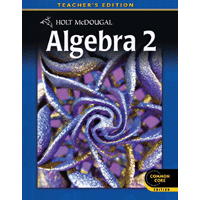# Alg 2 Quiz 4.1

25 Questions | Total Attempts: 323SettingsSections 4-1, 4-3, and 4-4

• 1.
Write  in logarithmic form
• A.
• B.
• C.
• D.

Not here

• 2.
Write the given logarithmic equation in exponential form.
• A.
• B.
• C.
• D.

Correct exponential form is not here

• 3.
Condense the given logarithmic expression into a single logarithm.
• A.
• B.
• C.
• D.

Correct form is not here

• 4.
Rewrite the logarithmic expression as a single logarithm.
• A.
• B.
• C.
• D.

Correct form is not here

• 5.
Rewrite the exponential equation in logarithmic form.
• A.
• B.
• C.
• D.

Correct form is not here

• 6.
Write the given exponential equation in logarithmic form.
• A.
• B.
• C.
• 7.
Write the given exponential equation in logarithmic form.
• A.
• B.
• C.
• 8.
Write the given exponential equation in logarithmic form.
• A.
• B.
• C.
• 9.
Write the given logarithmic equation in exponential form.
• A.
• B.
• C.
• 10.
Write the given logarithmic equation in exponential form.
• A.
• B.
• C.
• 11.
23.  Write the given logarithmic equation in exponential form.
• A.
• B.
• C.
• 12.
The Allen Electric Company has a piece of machinery valued at \$60,000.  It depreciates at 20% per year.  After how many years will the value have depreciated to \$15,000?  Use .
• A.
• B.
• C.
• D.

Not here

• 13.
Tony purchased a rare 1959 Gibson Les Paul guitar in 2000 for \$12,000.  Experts estimate that its value will increase by 12% per year.  After how many years will the value have increased to \$40,000?  Use .
• A.
• B.
• C.
• D.

Not here

• 14.
Tonya invests \$5000 in an account that pays 5% interest per year.  After how many years will her investment be worth \$8000?  Use .
• A.
• B.
• C.
• D.

Not here

• 15.
The Allen Electric Company has a piece of machinery valued at \$45,000.  It depreciates at 20% per year.  After how many years will the value have depreciated to \$15,000?  Use .
• A.
• B.
• C.
• D.

Not here

• 16.
Using the properties of logs, write in expanded form:
• A.
• B.
• C.
• D.

Not here

• 17.
Using the properties of logs, write in expanded form:
• A.
• B.
• C.
• D.

Correct form is not here

• 18.
Express as a single logarithm:
• A.
• B.
• C.
• D.

Not here

• 19.
Which function represents exponential decay?
• A.
• B.
• C.
• D.
• E.

None of these is exponential decay

• 20.
Which function represents exponential growth?
• A.
• B.
• C.
• D.
• E.

None of these is exponential growth

• 21.
Which ordered pair lies on the graph ?
• A.
• B.
• C.
• D.
• 22.
Rewrite the given logarithmic expression as a single logarithm.
• A.
• B.
• C.
• D.
• 23.
The expression  can be written as:
• A.
• B.
• C.
• D.
• 24.
If , then  is equivalent to:
• A.
• B.
• C.
• D.
• 25.
The expression  can be written as the single logarithm:
• A.
• B.
• C.
• D.
Related Topics